Posted Feb. 28/2020

Part X

The Big Bang: Visible Matter and DarkMatter

Compressible Flow and Thermodynamic Equations of State:

Exploring  the Possibility that Visible Matter May Have Originated at a Big Bang Embedded

Compressible Shock

and

The Dark Matter at a Subsequent Inflation Embedded Rarefaction Shock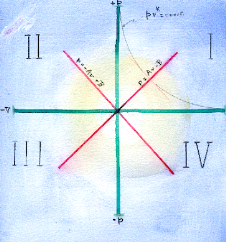Summary

In physical cosmology, the universe is known to contain about 4.9% visible matter, 27% dark matter and 68% dark energy. The visible matter is thought to have originated at the Big Bang explosion some 13.9 billion years ago.

Here, we explore the possibility that the concept of the compressibility of energy and its associated shock wave forms might  supply a unified physical framework capable of theoretically uniting at least two of   disparate main  components of our  universe and describing their emergence in a similar  process at or close to the Big Bang.

Visible matter is presented as originating at an intense compression shock associated with  the Big Bang.

The origin of the dark matter and its nature are  currently almost unknown, but here we explore its possible origin in a rarefaction  shock developing from and embedded in the super-inflation that immediately followed  the Big Bang.

Our approach is tentative, even sketchy, but seems adequately supported to warrant further examination.

[The dark energy is considered to constitute a vacuum energy density required to explain the known various quantum wave fields.   It is, however,  thought to have emerged when the universe was about half its present age, that is at about 7 billion years before present. It was introduced to explain an observed acceleration of the rate of expansion of the universe beginning at that time. From this point of view it would not be associated with the Big Bang, but may be associated with a Vacuum Energy Density, or with some later cosmological event, or may even eventually be seen as unnecessary to postulate at all.]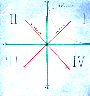Contents

1 Introduction

2.  Compressible Flow, Waves, Shock Waves, Shock Energy Condensations and Rarefactions

3. Evidence for he Origin of Baryonic Matter by Energy Compressibility in Shock Wave Condensations in Linear Accelerators

4.  Origin of our Visible Matter Via the Big Bang at Possible  Strong and Weak Compression Shocks, and the Origin of the ‘Dark Matter’ at the Big Bang Inflation as  Strong and Weak Rarefaction Shocks

• A New Big Bang Proposal

•  Proposed Elliptical Equation of State for Dark Matter

• How A Physical Compression Shock Model followed by  a Rarefaction Shock  Model Might Fit into the Standard Model of The  Big Bang

5. Some Relevant Further Questions to be Addressed

6. Conclusions

Appendix  A:  Outline of Compressible Fluid Flow

Appendix B: The Origin of Visible Matter in Linear Accelerators at  Strong and Weak Compressive Shocks

References1. Introduction

In a previous Page [ www.energycompressibility.infoPart VII: Equations of State for Cosmic Fields’] we explored the cosmological application of thermodynamic equations of state. Specifically, we proposed the  hyperbolic equation of state [ pv = constant]  for our visible matter in the  cosmos, the elliptical equation  [ p2/b2 + v2/a2 =  a2 b2 \ for the dark matter, and the linear equation [ p =  ± Av ] for stable waves of both types and possibly also for quantum electromagnetic waves. [ Fig. 1.].

As we shall show, the elementary particles of our visible  universe can  be described by  compression shocks in  the hyperbolic  state [pv = constant]. The evidence for this is that it  fits the  Standard Model ‘s data for elementary particles  formed in linear accelerators.

We here propose that the dark  matter’s elliptical  state, should  produce rarefaction forms, and that this rarefaction  may  explain both the dark matter’s  invisibility  and its non-interaction with other forms of matter, except by way of gravity.

The linear equation of state is taken as describing sets of linear  waves both of compression and of rarefaction. These may be associated with  electromagnetic waves.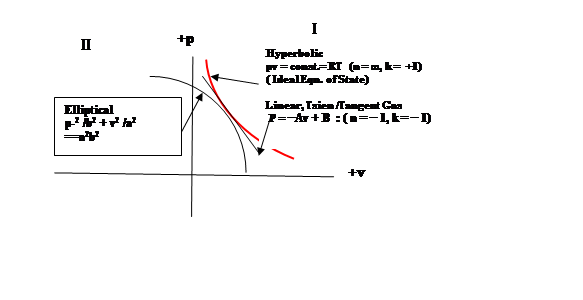Figure 1:  Equations of State for Visible Matter, Dark Matter and Linear Waves

The  ‘dark matter’ of the universe constitutes  about 28% of the total mass, which is  over five times as much  as that of our ordinary visible matter of  the Hubble cosmos [ 4.9 % ] . Its nature is still  almost unknown.. It gravitates, which is why we know it exists, but otherwise it apparently interacts very little, if at all, with the other constituents of the cosmos..

The concept of the ‘dark’ energy is mainly needed for its negative pressure which would account for the  increased rate of expansion of the universe recently discovered.   It acts through gravity and constitutes  about 68% of the mass energy of the entire cosmos. It is a successor  to  Lambda (Λ), the cosmological constant of general relativity, and to the vacuum energy density or e quantum vacuum energy, which are  all necessary  (1) to counteract the inward pull of gravity and so  prevent a collapse of the cosmos, and (2)  to account for the  acceleration in the rate of expansion observed since the 1990’s.. The dark energy is thus the latest in a succession of adjustments to the basic standard model pf physical cosmology.

[The dark energy is considered to constitute a vacuum energy density required to explain the known various quantum wave fields.   It is, however,  thought to have emerged when the universe was about half its present age, that is at about 7 billion years before present. It was introduced to explain an observed acceleration of the rate of expansion of the universe beginning at that time. From this point of view it would not be  associated with the Big Bang, but may be associated with a Vacuum Energy Density, or with some later cosmological event.]

We shall take the origin of the Hubbble cosmos  as being well described by the Big Bang Theory. In this, the visible or Hubble universe is described as originating from a state of extreme compaction of energy followed by a very short  inflationary interval  in which , as the  universe cooled by the immense expansion, the  elementary particles of matter formed. In the far slower expansion during the 13.9 billion years  which followed the Big Bang and the brief inflation, the astronomical universe of gas, nebulae, galaxies and stars gradually emerged.

Here we outline a proposal that the dark matter of the universe emerged in the Big Bang along with the  visible matter, the visible matter emerging in a compressible energetic flow governed by hyperbolic compression in the formation of baryons and leptons, while the dark matter is here theorized to have formed via an intense  rarefaction shock occurring in an elliptical state expansion and rarefaction flow in the inflationary period following the Big Bang compression.

Many questions arise, some of which we shall attempt to show can be explained by compressibility concepts. For example :  Why does the dark matter not interact with our visible or ordinary matter, nor with electromagnetic radiated waves?  Why do the three states depicted in Fig. 1 at their  common tangent point, not interact there?  Again, can the compression  shock proposed as forming the visible universe’s elementary particles of matter be identified with the Big Bang’s  intense compression state? And can the proposed rarefaction shock for the formation of the dark matter  be identified with the termination of the  Big Bang inflationary period?

Since our answers will involve waves and shock waves, we shall briefly outline some of these effects.2.  Compressible Flow, Waves, Shock Waves, Shock Energy Condensations and Rarefactions

There are two possible types of stable pressure waves, namely compression waves and rarefaction waves. If these are of low amplitude, they are stable and are called acoustic type waves. If they are of finite amplitude they are unstable, giving us, in the hyperbolic case of our visible matter, compression waves which grow quickly to form compression shocks. In the elliptical state case they  appear as rarefaction waves,  growing quickly  to form rarefaction shocks.

This prompts us to ask: What happens  if rarefaction waves of one state encounter compression waves of another state, say elliptical waves ( rarefaction) encountering hyperbolic (compression)  waves) ?  An intuitive answer is that they might  act to nullify one another. If so, does this explain why the dark matter (elliptical and rarefied) does not apparently interact with our visible world matter (hyperbolic and compressed)? Or is the answer that they are just too dissimilar to interact ( except through gravity).

First, for clarity,  we shall briefly review some pertinent aspects of compressible flow theory.Compressible Flow

Compressible fluids can undergo increases in pressure ( compression) or decreases in pressure ( rarefaction or expansion). These pressure changes are accompanied by reciprocal changes in specific volume ( volume per unit mass).

The thermodynamic variables of pressure p, specific volume v = 1/ρ,  and temperature T are interrelated, and are given by an equation of state (  e.g. pv = RT, the common hyperbolic gas law in our visible matter world).Waves in Compressible Flow

Pulses or changes in flow rate in compressible fluids cause pressure fluctuations of compression or rarefactions which result in traveling disturbances called   pressure waves.  These can be of either compression or rarefaction depending on the physical nature of the compressible fluid. Waves in material gases of visible matter are always compression while  rarefaction waves are damped and die out.

If the wave amplitude is ‘infinitely’ small, the waves are  stable and are clled ‘acoustic waves’. If the wave amplitude is finite to begin wit,h then the waves grow rapidly to form shocks or shock waves.

The criterion for wave behavior is the curvature of the equation of state d2p/dv2 on the pv diagram. If the curvature is positive              [ d2p/dv2 › 0] as in the hyperbolic equation of state of material gases then hyperbolic compression  waves are non-linear and steepen to shocks and rarefaction waves die out.

If the curvature is negative [d2p/dv2 ‹ 0], as in the elliptical dark  matter, then their rarefaction waves are non-linear and  steepen with time to form rarefaction shocks while compression waves die out.

[Note: In all cases, if the pressure disturbances are of infinitesimally low amplitude then stable waves of either compression  or rarefaction  called acoustic waves can persist without growing to shocks].

In the case where the curvature is zero [d2p/dv2 = 0],  the pv curve is a straight line and waves of both compression or rarefaction, and of any amplitude large or small, are all stable and can propagate unchanged. Growth to shock waves is not possible in the linear case.Shock Waves

With both hyperbolic  and elliptical ( Fig.1) equations of state,  finite amplitude perturbations grow to form shocks. These are highly concentrated states through which, along the directions of flow,  the pressure p, tempersture T and specific volume v, change . The magnitude  of these changes in these state variables through the shock discontinuity is directly related to shock strength.

Shocks can be perpendicular to the direction of  flow  ( i.e. ‘normal’ shocks0 or oblique to the flow ( oblique shocks).

For more detail on shocks see Appendix A : Outline of Compressible Flow Physics.

All finite amplitude, compressive waves are non-linear and grow in amplitude with time to form shock waves.  These shocks  are discontinuities in flow, across which the flow variables p, ρ, V, T and c change abruptly. (Note p = pressure).Normal Shocks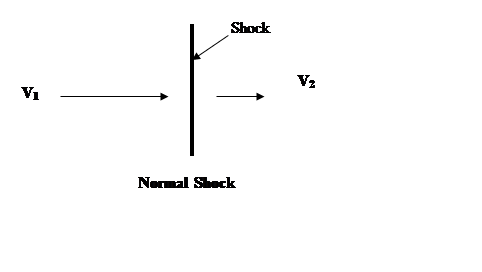V1  >  V2                                                                                          (8)

p1, ρ1, T1   <  p2, ρ2,  T2                                                                              (9)Entropy Change Across Shock:

∆S = S1 – S2 = − ln(ρ0201)                                                      (10)Maximum Condensation Ratio:

ρ12 = [n+1]1/2 = Vmax/c*                                                   (11)Oblique Shocks

If the discontinuity is inclined at angle to the direction of the oncoming or upstream flow, the shock is called oblique.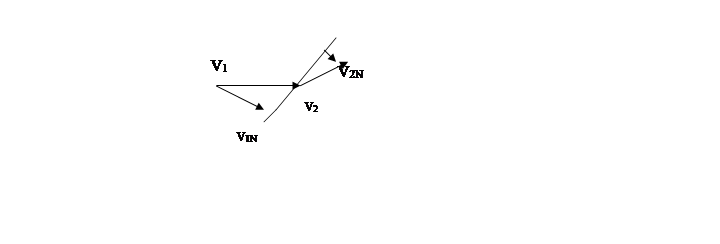Oblique Shock

V1N  >  V2N

p1ρ1T1  <  p2ρ2

Since the flow V is purely relative to the oblique shock front, the shock may be transformed to a normal one by rotation of the coordinates, and the equations for the normal shock may then be used instead.Equations of State

These refer to equations linking the thermodynamic state variables of pressure, p,  specific volume (v = 1/ρ) and temperature T.

For example, we have the Ideal Gas Law as the hyperbolic   equation of state for visible matter[ pv = const. = RT]; and the elliptical equation iof state  [p2/b2 + v2/a2 = a2b2 = const] which we here propose to describe the dark matter of the cosmos.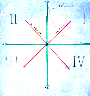3. Evidence for  the Origin of Baryonic Matter by Energy Compressibility in Shock Wave Condensations

We propose that : All elementary particles of matter (with the possible exception of the neutrino) are condensed energy forms produced under hyperbolic equations of state by compression  shocks.[1,2,3].

The forms are given in terms of a simple, integral number n  ( n = degrees of freedom of the compressible energy flow,  which is roughly the number of ways the energy of the system is divided)..  The experimental values of the ratio of the masses to one another are then related  to the maximum theoretical compression ratio for each compression shock. ( Eq. 16 below).  The observed fit is to within 1%.

A. Formation of  Baryons and Heavy Mesons) in a Linear Accelerator

Maximum Compression Ratio

mb/mq = Vmax/c* = [n+1]1/2                                                                                                                       (16)

mb is the mass of any hadron  particle, mq is a quark mass, Vmax = co n1/2 is the escape speed to a vacuum; that is, it is the maximum possible relative flow velocity in an energy flow for a given value of n, the number of degrees of freedom of the energy form,  This is a non-isentropic relationship which  corresponds physically to the maximum possible strong shock.

.

Experimental verification values for  this hadron mass- ratio formula are given in Table  A below. (See Appendix  B: The Production of Visible Matter at a Strong Condensation Shock in a Linear Accelerator )

Table A)  Hadrons (Baryons and Heavy Mesons

--------------------------------------------------------------------------------------------

n     n +1     [n+1]1/2    Particle         Mass (mb)        Ratio to

( MeV)            quark mass

_____________________________________________

0     1             1          quark (ud)         310 MeV          1

quark (s)            505

1

2     3             1.73      eta (η)                548.8               1.73

3

4

5     6             2.45       rho (ρ)               776                 2.45

6

7

8     9             3          proton (p)           938.28          3.03  (1)

neutron (n)          939.57          3.03

Λ  (uds)             1115.6            2.97  (2)

Ξo (uss)             1314.19          2.99  (3)

9   10           3.16      Σ+  (uus)           1189.36          3.17  (2)

10   11         3.32      Ω-  (sss)            1672.2            3.31  (4)

Note: Average quark mass is 310 MeV;  (2) Average quark mass is (u + d+ s)/3 = 375 MeV   (3) Average quark mass is (u+s+s)/3 = 440 MeV;   (4) Average quark mass is 505 Mev.

Comparing column three,  the maximum shock compression [n+1]1/2 ], to the final column  “Ratio to quark mass” we see that they closely agree,  so that the proposed origin of hadrons by strong shock compression theory expressed  in Equation 16 is verified.

B. Origin of  Leptons, Pion and Kaon

mL/me-  =  k/α2 = [(n+2)/n]/α2 = {(n+2)/n] x 137                                                 (17)

where α = 1/11.703  = [1/137]1/2 is the fine structure constant of the atom , and k is the adiabatic exponent or ratio of specific heats, k = cp/cv = [(n+2)/n].

Because of the presence of k, this equation for the mass of the leptons is  thermodynamic and quasi-isentropic.

We propose that the leptons are formed via the weak shock option( i.e. they involve the reduction in strength of the fine structure constant [1/137]1/2

The experimental verification for the lepton mass ratio formula of Eqn. 17 is given in Table B below.

Table B)  Leptons, Pion and Kaon

a

N     k = (n+2)/n       Particle                  Mass              Ratio        Ratio

(MeV)              to              x 1/137

Electron

__________________________________________________________

1/3           7              Kaon  K±               493.67            966.32          7.05

2              2              Pion π±                  139.57            273.15          1.99

4              1.5           Muon μ                  105.66            206.77          1.51

-                -             Electron                 0.511              1

Clearly, column 2 values for  k ≈ ml/me (1/137)  closely match column 6 for the mass ratio reduced by 1/137, thus verifying  Equation 17 and the theory that the leptons are formed by weak shock condensation. .

Summary

The problem of the origin of the observed mass-ratios of the elementary particles of matter to one another has here been  explained by the compressible flow expressions to within about 1% of the experimentally observed values. This grounds the creation of matter in the strong compressible shock for the baryons, and in the weak shock option for the electron and leptons.

The principle of the compressibility of energy flow, therefore, would seem to underlie the emergence of  material particles in  the  visible  universe from some underlying energy field or continuum such as a modified  general relativity field.

The above data are those of the standard model of elementary particle formation as verified in high energy accelerators. Next we propose that this shock compression process for particle formation in accelerators may fit into the existing Big  Bang model as well.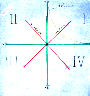4. Origin of Visible Matter of the Cosmos Via the Big Bang in  Strong and Weak Compression Shocks, and the Origin of the ‘Dark Matter’   Via  the Subsequent  Inflation  in  Strong and Weak Rarefaction ShocksA New Big Bang Proposal

The Big Bang theory for the origin of the material, visible universe of baryons and leptons is acknowledged to be a  very successful explanation.

The nature and origin of other constituents of the cosmos, such as the dark matter and dark energy, are today still open questions.

Therefore, we here are going to tentatively explore the possibility that the addition to the Big Bang explosion process of the compressible fluid flow, flow  waves and  shock wave processes, for both compressive and a succeeding rarefaction,  may provide for the origin of our visible matter and  for the origin of the dark matter as well.

r

In short, if compressible flow process can be integrated into the Big Bang event, then shock wave  condensation process can account for the visible natter, and so also, we now propose,  the subsequent rarefaction flow and rarefaction shock process could account for the emergence of the  dark matter, for its observed  ‘dark’ and non-interaction properties and possibly for its relative abundance aa well

A. Origin of Visible Matter

We have shown above how visible matter’s elementary particles, baryons and leptons, can be explained as  emerging from the reaction of a  liner accelerator by envisaging a   compressible flow’s strong and weak compression shocks.

We have now extended this approach to the origin of matter in the Big Bang’s intense explosion and the subsequent inflationary expansion.

Maximum Strong Shock Compression Ratio

mb/mq = Vmax/c* = [n+1]1/2                                                                                                                       (16)

mb is the mass of any hadron  particle, mq is a quark mass, Vmax = co n1/2 is the escape speed to a vacuum; that is, it is the maximum possible relative flow velocity in an energy flow for a given value of n, the number of degrees of freedom of the energy form,  This is a non-isentropic relationship which  corresponds physically to the maximum possible strong shock.

If the visible matter equation of state is taken as the Ideal Gas Law [ pv = constant],  then we note that,  the corresponding degrees of energy , freedom become theoretically infinite [ n = ∞  ]. Thus the maximum condensation ratio in this fluid would be , not infinite, but, practically speaking,  enormous. This enormous condensation ratio for the strong shock would correspond well to the enormous condensed state required at the initial instant of the  Big Bang Theory. Thus,  shock compressibility theory and Big Bang theory are in  agreement on  this requirement.

B. Origin of Dark Matter

Because , as we have shown, the dark’ matter’s elliptical equation of state requires rarefaction waves,  its’ particles’ must then be rarefied energy forms instead of the condensed energy forms of our ordinary visible matter.

We now propose that the dark matter may have originated via the  alternative   strong and weak rarefaction shocks which could have occurred in the expansion or rarefaction  period following on the Big Bang.

That is to say, the visible matter formed first at compressive shocks associated with the initial high pressure explosive phase of the Big Bang; subsequently  the rarefied, elliptical dark matter was  formed in the inflationary expansion /rarefaction phase of the Big Bang.

It would seem that this dark matter proposal of ‘rarefaction forms’ instead of our visible matter’s ‘condensed forms’ ,could now account for (1) its  invisibility  (2) its weak or non-existent interaction with visible matter and with electromagnetic radiation. (3) its relative abundance may be related.

In addition, it may also  provide some insight into the reason for the  dark matter’s relative abundance being some 5 times greater than our visible cosmic matter, namely 27% of the  total  mass energy of the cosmos  versus only 4.9%  for our  visible matter. One may ask questions such as: Q.   Is its abundance related to the much larger volume of space available  for any cosmic event in the expansion after the Big Bang as compared with the tiny volume assigned to thr actual Big Bang event ‘s highly condensed state.

taking place

IProposed Elliptical Equation of State for Dark MatterHow A Physical Compression Shock Model followed by  a Rarefaction Shock  Model Might Fit into the Standard Model of The  Big Bang

First, a caveat:  Our proposal ,which follows, that  compressible shock phenomena may be , intimately involved in the standard Big Bang  cosmology, is necessarily tentative and suggestive only

Its justification appeals to the correlation evidence given above for shock compressions being involved in elementary particle production processes in linear accelerators. We note  that the detailed complexities of such applications are a matter for the specialists.

1. The ‘super-strong strong shock would fit in at the current initial maximum density and tempersture Planck epoch.  The baryon and lepton matter would emeege or form there.

2. The inflationary epoch would seem to fit in with  post strong shock, ‘down stream’, expansion flow.

3. A super rarefaction weak shock episode might then terminate the inflationary expansion epoch. The seeds of the dark matter ( which we postulate to be rarefaction forms)  would emerge or  form in this expansion.

This Big Bang intense compression is  immediately followed by an intense inflationary expansion or rarefaction which could bring about  an intensely strong rarefaction shock. In such a rarefaction shock, immediately following the Big Bang condensation, we speculate that the dark matter forms.  [ Since the volume enclosed and the surface area of the rarefaction shock are larger than the big bang compression sphere, therefore the amount of dark matter formed by this succeeding rarefaction, one could speculate, could be  greater than the visible matter formed from the  big bang condensation.   Conceivably then    this “ big bang” double process of  of compression and subsequent rarefaction may helpful in an explanation for the  observed 25% to/4.9%  ratio of dark matter mass to visible universe matter seen at the present day.

These proposals are clearly suggestive only, and a convincing account would  require the expertise and cooperation of compressible flow physicists with  cosmologists.

Maximum Compression Ratio

mb/mq = Vmax/c* = [n+1]1/2                                                                                                                       (16)

mb is the mass of any hadron  particle, mq is a quark mass, Vmax = co n1/2 is the escape speed to a vacuum; that is, it is the maximum possible relative flow velocity in an energy flow for a given value of n, the number of degrees of freedom of the energy form,  This is a non-isentropic relationship which  corresponds physically to the maximum possible strong shock.

If the visible matter equation of state is taken as the Ideal Gas Law [ pv = constant],  then we note that,  the corresponding degrees of energy , becomes theoretically infinite [ n = ∞  ]. Thus the maximum condensation ratio in this fluid would be, certainly not infinite  but, practically speaking,  speaking, certainly enormous. This enormous condensation ratio for the strong shock would correspond well  the enormous condensed state required at the initial instant of the  Big Bang Theory. Thus,  shock theory and Big Bang theory are in  agreement on  this requirement.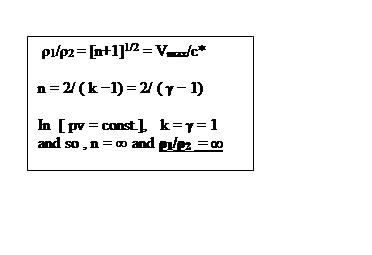5. Some Specific Further Relevant Questions to be Addressed

1. Does the proposed compressive shock fit time- wise  before, or in, or after the Big Bang?  Can the new proposal  solve this  ?

2. At what stage after the Big Bang would the rarefaction shock and dark   matter formation best fit?

3. Can we now better explain the 5 to 1 rstio of dark matter  to visible matter, e.g. 27%/4.9%.

4.  Does the dark energy fit in here at all?  It is usually invoked to explain an  observed increased rate of expansion of the cosmos about

half way in time between the Big Bang and the present time which is to say around 7 billion years age. Currently an energy density amounting  to around 65% of the total is required [ 65%27%4.9%

5. Compressible flow theory requires a flow of “something” in which waves and shocks form.  Is this ‘something’ a ‘vacuum energy density’ ?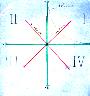6. Conclusions

We have proposed that imposition of compressible flow theory  into the Big Bang hypothesis produced many points of explanation of current cosmic cosmological mysteries, for example:

The immense original matter energy density of the Big Bang  is in agreement with a  hyperbolic ideal fluid strong shock

compression.

The emergence of the elementary particles of matter of quantum theory is compatible with the same strong shock process.

The dark matter can b envisaged as an elliptical rarefaction wave flow occurring in conjunction with the period of hyperinflation following the  Big Bang. In addition  ‘elementary particles of dark matter can be  predicted to form from a rarefaction shock process  occurring in this standard hyperinflationary episode.

Because compressible theory predicts dark matter would be  rarefaction in nature, this this would intuitively also  be expected not to interact with compressed visible  matter and  radiation. This non-interaction is what is observed and which at present cannot be explained.. Explaining this mystery  would be  string point in favor of the new compressible approach.

The observed relative amounts of visible (4.9%) and dark matter (28 %) can possibly also now be explainable.

. The baryons and leptons of visible matter can be related to condensation shocks in a compressible  flow.

First, this suggests that the origin of visible matter in the Big Bang condensation nay have involved the shock compression process.

Second, if the dark matter has an elliptical equation of state, then its forms will  be  rarefied  and would form  rarefaction  shocks. This further suggests that the  rarefied dark matter may have firmed in such rarefaction shocks set off by the sudden cosmic inflationary stage which immediately followed the Big Bang condensation.

The lack of interaction of the dark matter with visible matter may be due to the fact that it is a rarefied form, whereas visible matter is made up of condensed forms.

The invisibility of the dark matter may be explained in a similar way by noting that rarefied matter may not interact with compressible leptons such as electrons and photons.

Dark matter forms and rarefied waves should interact with each other.

The degree of condensation or compression of the Big Bang event was enormous.  This is matched by compressible theory in the case of the Ideal Equation of State [pv = const.] where the maximum condensation of its str0ong shock is infinite. We have,

Maximum Condensation ratio is:    mb/mq = [n+1]1/2

And so, in  pv = const we have k = 1 and therefore n, the energy division becomes infinite.[ n = 2 / (k-1  = 2 / 0 = ∞ ] Therefore,  the maximum condensation ratio also becomes infinite.

Thus, the Big Bang’s extreme condensation state is compatible with compressible theory for the case of The Ideal  Equation of  State.  This agreement seems quite remarkable, and it provides powerful support for the compressible theory’s application to Big Bang theory.

References

1. Power, Bernard A., Unification of Forces and Particle Production at an Oblique Radiation Shock Front. Contr. Paper N0. 462. American Association  for the Advancement of Science, Annual Meeting,  Washington, D.C., Jan. 1982.

2. .---------------, Baryon Mass-ratios and Degrees of Freedom in a Compressible Radiation Flow.  Contr. Paper No. 505. American Association for the Advancement of Science, Annual Meeting, Detroit, May 1983.

3. .---------------, Summary of a Universal Physics. Monograph (Private distribution) pp 92.  Tempress, Dorval, Quebec, 1992. ( Appendix B: Summary of a Universal Physics”)

4. . Shapiro, A. H. The Dynamics and Thermodynamics of Compressible Fluid Flow.  2 vols. John Wiley and Sons, New York, 1953

5.  Courant, R. and Friedrichs, K. O. (1948). Supersonic Flow and Shock Waves.  Interscience, New York.

6. Lamb, Horace., Hydrodynamics    6th ed. Dover, New York, 1932.

7. Chaplygin, S.,  Sci. Mem. Moscow Univ. Math. Phys. 21 (1904).

8. Tsien, H. S.  Two-Dimensional  Subsonic Flow of Compressible Fluids,  J. Aero. Sci. Vol. 6,  No.10 (Aug., 1939),  p.  399.

9. Bachall, N.A., Ostriker, J.P., Perlmutter, S., and P.J. Steinhardt. The Cosmic Triangle: Revealing the State of the Universe. Science, 284, 1481 1999.

10. Kamenshchick, A, Moschella, U., and V. Pasquier. An alternative to quintessence. Phys. Lett. B 511, 265, 2001.

11. Bilic, N., Tupper, G.B., and R.D. Violier. Unification of Dark Matter and Dark Energy: The Inhomogeneous Chaplygin Gas. Astrophysics , astro-ph/0111325. 2002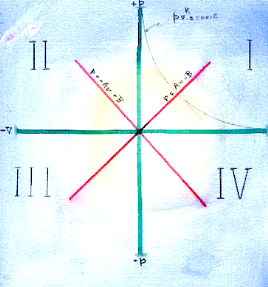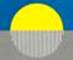Copyright,  Bernard A. Power,  Feb. 2020

Appendix A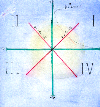2.0 Outline of Compressible Fluid Flow

The following is a listing of some relevant  basic  compressible flow principles. For  more complete treatment see  texts on compressible fluid  flow or  gas dynamics.[2.1  Steady State Energy Equation

c2 = co2    V2/n                                                                              (1)

where c is the local wave speed, co is the static [i.e at V = 0] or maximum wave speed, V is the relative flow speed, n is the number of ways the energy of the flow system is divided (i. e.  the number of degrees of freedom) of the system and [  n = 2/(k− 1),  where k = cp/cv   is  the’ adiabatic constant’  or ratio of specific heats.]

Here, ‘relative’ means referred to any (arbitrarily) chosen physical flow  boundary. The equation is for unit mass, that is, it pertains to ‘specific energy’ flow.

The case where V = c = c*  is called the critical state. The ratio (V/c) is the Mach number M of the flow.  The ratio (V/co) is also  a quantum state variable. The maximum flow velocity Vmax (when c = 0)  is the escape speed to a vacuu:

Vmax = √ n co.2.2   Unsteady State Energy Equation

c2 = co2 – V2/n – 2/n (dφ/dt)                                                                         (2a)

where φ is a  velocity potential, and dφ/dx = u is a perturbation of the relative  velocity.  Therefore, in three dimensions, substituting V for u, we have dφ/dt = V (dx/dt) = Vc, and

c2 = co2 – V2 /n  − 2 (cV)/n                                                                      (2b)

(See also sect. 3.2 below  for far-reaching implications of the 2cV interaction energy term in quantum state physics).2.3  Lagrangian Energy Function L

L = (Kinetic energy) – (Potential energy) = ( c2+ V2/n) – co2 , and so, from (2b)

L = − (2cV)/n                                                                                         (3)2.4  Equation of State for Compressible,  Ordinary Matter Systems

The  equations of state  link the thermodynamic quantities of pressure p, specific volume ( volume per unit mass v = 1/ρ] and temperature T. The basic equation of state for ordinary gases is the equilateral hyperbola of the Ideal Gas Law:

pv = RT ;     p/ρ = RT = constant                        (4)

Equation 4 is seen to be isothermal  (T= constant) . For adiabatic changes it becomes pv­k = constant, where the adiabatic constant  k = cp/cv is the ratio of the specific heats at constant pressure  and at constant volume,  respectively.

Here, each point on the curve presents the values for a particular  pressure and volume pair  and shows how the two relate to each other when one or the other is changed.  In this hyperbolic equation, the product of the two -- i.e. the  pv- energy --  has a constant value as set out by the equation of state.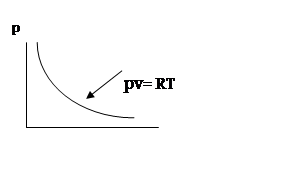Hyperbolic Equation of State (Ideal Gas Law)

Equations of State  can be formed for gases, liquids or solids. Here, we shall be concerned mainly with those for the highly compressible states i.e. for  gases.2.5     Waves and Flow

2.5.1 The  Classical Wave Equation

2ψ = 1/c2 [∂2ψ/∂t2]                                                                   (5)

where ∆2 = ∂2../∂x2  + ∂2.../∂y2 + ∂2../∂z2;  ψ  is the wave amplitude, that is, it is the amplitude of a thermodynamic state variable such as the pressure p or the density ρ. The local  wave speed is c.

The general solution of (5) is

ψ = φ1 (x – ct) + φ2 ( x+ ct)                                                                         (6)

Equation 6 is a linear,  approximate  equation for the case of low-amplitude waves in which all small terms (squares, products of differentials, etc.) have been dropped.

The natural graphical representation of steady state compressible flows and their waves is on the (x,t), or space-time diagram.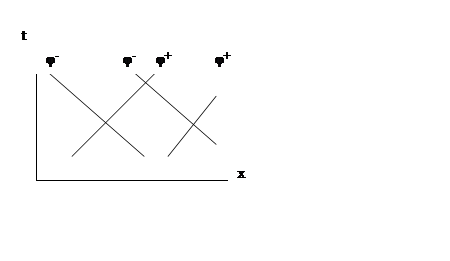The classical wave equation corresponds to isentropic conditions. It represents a stable, low-amplitude wave disturbance, such as an acoustic–type wave.Unsteady State  ( Accelerating) Compressible Flow

In compressible flow theory, forces, when present,  introduce a curvature of the characteristic lines for velocity on the space-time or xt-diagram. Space- time curvature thus indicates velocity acceleration and the presence  of force .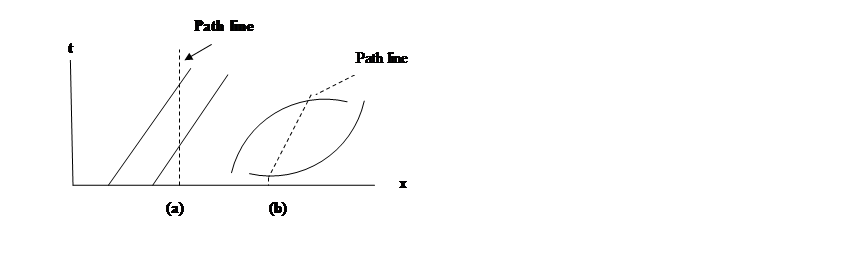(a) Straight characteristics and path lines show steady flow and absence of force.

(b) Curved characteristics and path lines show acceleration and presence of force

In the case of compressible flow and 3-space (x,y,z) ,  a curved path line dx/dt = v (path) may be ‘transformed away’ to a straight line Lagrange representation [ dh/dt = 0].

[Note:  In General Relativity the analogous distortion of its 4-space (x,y,z,t) to obtain a force- free representation is a tensor distortion.

However, it should be noted that general relativity is a continuous field theory, and, as such, excludes discontinuities or singularities such as shocks. Therefore, it appears to be fundamentally incompatible with quantum physics.

On the other hand, compressible flow as shown below in Section 3.1 on Visible Matter predicts shock discontinuities as the physical mechanism for the emergence of the elementary particles of matter by shock compression of an energy flow.  Thus, compressible flow is compatible with quantum theory whereas general relativity is not.]2.5.2     The Exact Wave Equation

Ñ2 ψ  = 1/c22ψ/∂t2 [ 1 + Ñψ ](k + 1)                                                            (7)

where k, the adiabatic exponent, is k = cp/cv = ( n + 2) /n; and ( k + 1 ) = 2( n + 1)/n =  2(co/c*)2.   Here, pressure is a function of density only.  This wave is isentropic, non-linear, unstable, and grows to a non-isentropic discontinuity called a shock wave.2.5.3     Shock Waves

All finite amplitude, compressive waves are non-linear and grow in amplitude with time to form shock waves.  These shocks  are discontinuities in flow, across which the flow variables p, ρ, V, T and c change abruptly. (Note p = pressure, ρ  = momentum).2.5.3.1     Normal ShocksV1  >  V2                                                                                          (8)

p1, ρ1, T1   <  p2, ρ2,  T2                                                                              (9)Entropy Change Across Shock:

∆S = S1 – S2 = − ln(ρ0201)                                                      (10)Maximum Condensation Ratio:

ρ12 = [n+1]1/2 = Vmax/c*                                                   (11)2.5.3.2     Oblique Shocks

If the discontinuity is inclined at angle to the direction of the oncoming or upstream flow, the shock is called oblique.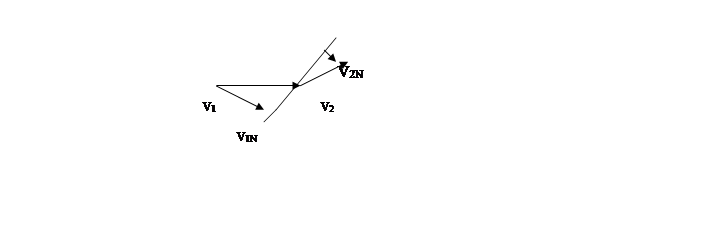Oblique Shock

V1N  >  V2N

p1ρ1T1  <  p2ρ2

Since the flow V is purely relative to the oblique shock front, the shock may be transformed to a normal one by rotation of the coordinates, and the equations for the normal shock may then be used instead.2.5.3.3  Strong and Weak Oblique Shock Options: The Shock Polar

For each inlet Mach number M1 ( = VN1/c), and turning angle of the flow θ, there are two physical  options:

1) the strong shock ( intersection S) with strong compression ratio and large flow  velocity reduction (p2 >> p1;  V2 << V1, or

2) the weak shock (intersection W, with small pressure rise and small velocity reduction.

Which of the two options occurs depends on the boundary conditions: low back i.e. low  downstream pressure favours the weak shock occurrence; high downstream pressure favours the strong shock.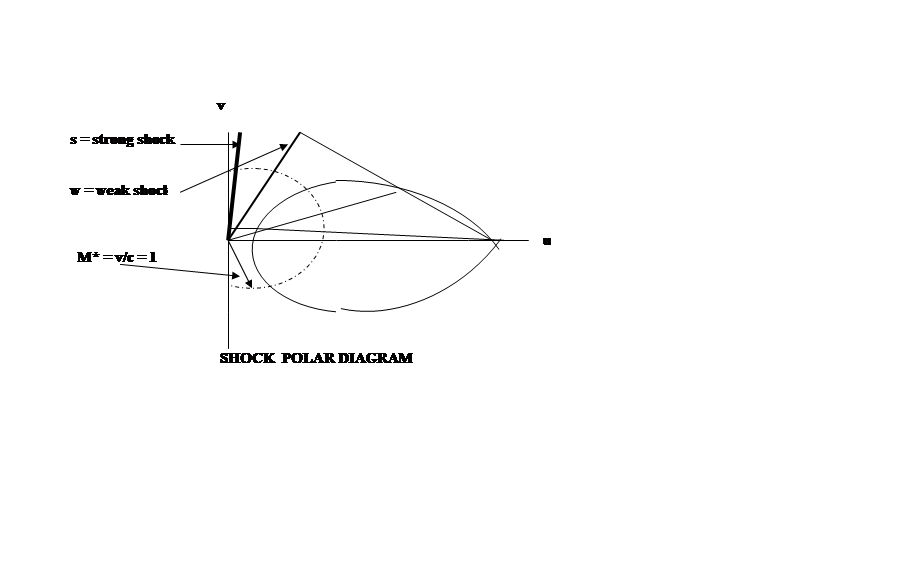When the turning angle θ of the oncoming flow is zero, the strong shock becomes the normal or maximum strong shock, and the weak shock becomes an infinitesimal, low-amplitude, acoustic wave.2.6   Types of Compressible Flow:

a) Steady, subcritical flow ( e.g. subsonic, V< c), governed by elliptic, non-linear, partial differential equations.

b) Steady, supercritical flow ( e.g. supersonic,  V1 > c) governed by hyperbolic, nonlinear, partial differential equations.

c) Unsteady flow  (either subcritical or supercritical). These are wave equations governed by hyperbolic, non-linear, partial differential equations. They are often simplified to linear approximations, for example to the classical wave equation (5); if of finite amplitude they grow to shocks..

The solutions to the above hyperbolic equations are called characteristic solutions.  If linear, they correspond to the eigenfunctions and eigenvalues of the linear solutions to the various wave equations of quantum mechanics ( Sect. 3.7), or, equally, to the diagonal solutions of the matrix equation of Heisenberg’s formulation of quantum mechanics.2.7 Wave Speeds

c = [ co2 – V2/n]1/2     (steady flow)                                        (12)

c = co2 – V2/n – [(2/n)cV ]1/2    (unsteady flow)                         (13)

c2 =  (dp/dρ)s, where s is an isentropic state.

Since V is relative, it may be arbitrarily set to zero to give a stationary or “local” coordinate system moving with the flow; this automatically puts c = co and transforms the variable wave speed to any other relatively moving coordinate system.

The shock speed U is always supercritical (U > c) with respect to the upstream or oncoming flow V1.2.8 Wave Speed Ratio c/co  and The  Isentropic Thermodynamic Ratios

c/co = [1 -1/n(V/co)2]1/2  = (p/po)1/(n+2) = (ρ/ρo)1/n = (T/To)1/2                                              (14)

All the basic thermodynamic  parameters of a compressible isentropic flow are therefore specified by the wave speed ratio c/co. .2.9    Relativity Effects in Compressible Flow:   In compressible flows  all velocities [V or u] are relative only, and, moreover, the wave speed  c  is a variable which is  dependent on V and n; it decreases for larger velocities V. and it reaches its  maximum value co at the static state i.e. at  zero flow (V =0).

Interestingly, Equation (14)

c/co = [1 -1/n(V/co)2]1/2  = (p/po)1/(n+2) = (ρ/ρo)1/n = (T/To)1/2             (15)

shows that the  correction factor for the effect of flow speed on  wave speed c  on the right hand side of the equation has the same  form as the Lorentz Transformation of special relativity. If n = 1 the two correction factors become formally identical.

The differences from special relativity  are that the wave speed c is now a variable and a function of the flow velocity V,  and that there is the  energy partition constant  n.  Since the wave speeds are  low ( c =  334 m/s for air at m.s.l.),  the ‘Lorentz’   corrections for physical compressible systems such as  gases are relatively large. Also, the flow speeds can exceed the wave speed ( supersonic  flow),  whereas in special relativity theory, the wave speed c is a  constant ( 3 x 108 m/s) which  can never be exceeded.

Photon shocks are thus impossible in special relativity, whereas in compressible flow they furnish a quantum physical theory for the origins of matter itself via the  formation of the elementary particles of matter at  compression shock discontinuities.2.10  Wave Stability

Compression waves are the rule in the baryonic physical world ( i..e. in Quadrant I on the pressure-volume diagram) where  density waves are always compressive  and all compression waves of finite amplitude grow towards shocks.  Here, only acoustic compression waves ( i.e. infinitely low-amplitude compressions) are stable.

Finite rarefaction waves and rarefaction shocks are impossible in material gases;  only infinitely low-amplitude rarefaction waves can persist.

We shall see below that, with elliptical equations of state ( dark matter) and linear equations of state ( quantum radiation), stable, finite rarefaction waves do become possible.2.11   Elliptical Equations of State and Rarefaction Waves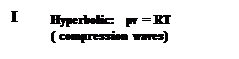II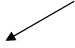+p-v

 +v

 -p

Elliptical Equation of State favours Rarefaction Waves and Shocks

The criterion for wave behaviour   is the curvature ( dp/dv)  of the isentropic equation of state:

1. If d2p/dv2 is > 0 (i.e. the  hyperbolic curve)  compression waves form  and steepen, while rarefaction waves flatten and die out.  Only compression waves of infinitely small amplitude (“acoustic” or “sound” waves” )  are stable.

2. If  d2p/dv2 is < 0 ,  ( i.e. the elliptical curve)  rarefaction waves form and steepen,  while compression waves flatten and die out.

.

We therefore see that  ordinary gases are hyperbolic and favour compression waves and  compression  shocks. No real gas is known whose  equation of state is elliptical and favours rarefaction waves;  but we are now proposing that it is this elliptical relationship which furnishes the Equation of State  for the  Dark Matter of the universe. ( Section 3.3 below).

We are also proposing that the third option , namely the  linear equation of state, fits the  Quantum state and the Electromagnetic field.  ( Section 3.2 below).

The Origin of Visible Matter at a Strong Compressive Shock in a LinearAccelerator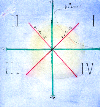3.1  Ordinary Visible Matter

Ordinary matter consists of elementary particles held together as atoms and molecules by electromagnetic forces.  Atomic and molecular matter can exist in three states as gas, liquid and solid. Gases are highly compressible and all the laws of compressible flow apply to them. Liquids and solids range from slightly compressible and  distortional to completely incompressible.  All three physical states can support waves. .

First, we examine the question of What is Matter?  Here we shall show that compressible flow theory gives a direct answer to this crucial question. Clearly, matter is formed from elementary particles.  But a deeper or more ultimate question is: How  do the elementary particles themselves arise?  Here we shall show that, if we start with energy as a compressible entity,  then the elementary particles can arise naturally from compressible theory as energy  shock wave condensations in a  compressible energy flow.A Theory of the Origin of Baryonic Matter: Energy Compressibility in Shock Wave Condensations

We propose that : All elementary particles of matter (with the possible exception of the neutrino) are condensed energy forms produced from hyperbolic equations of state by compression  shocks. .

The forms are given in terms of a simple, integral number n  ( n = degrees of freedom of the compressible energy flow,  which is roughly the number of ways the energy of the system is divided)..  The experimental values of the ratio of the masses to one another are then related  to the maximum theoretical compression ratio for each compression shock. ( Eq. 16 below).  The observed fit is to within 1%.

A. Origin of Hadrons (Baryons and Heavy Mesons)

Maximum Compression Ratio

mb/mq = Vmax/c* = [n+1]1/2                                                                                                                       (16)

mb is the mass of any hadron  particle, mq is a quark mass, Vmax = co n1/2 is the escape speed to a vacuum; that is, it is the maximum possible relative flow velocity in an energy flow for a given value of n, the number of degrees of freedom of the energy form,  This is a non-isentropic relationship which  corresponds physically to the maximum possible strong shock. .

Experimental verification values  this hadron mass- ratio formula is given in Table  A below.

Table A)  Hadrons (Baryons and Heavy Mesons)

--------------------------------------------------------------------------------------------

n     n +1     [n+1]1/2    Particle         Mass (mb)        Ratio to

( MeV)            quark mass

_____________________________________________

0     1             1          quark (ud)         310 MeV          1

quark (s)            505

1

2     3             1.73      eta (η)                548.8               1.73

3

4

5     6             2.45       rho (ρ)               776                 2.45

6

7

8     9             3          proton (p)           938.28          3.03  (1)

neutron (n)          939.57          3.03

Λ  (uds)             1115.6            2.97  (2)

Ξo (uss)             1314.19          2.99  (3)

9   10           3.16      Σ+  (uus)           1189.36          3.17  (2)

10   11         3.32      Ω-  (sss)            1672.2            3.31  (4)

Note: Average quark mass is 310 MeV;  (2) Average quark mass is (u + d+ s)/3 = 375 MeV   (3) Average quark mass is (u+s+s)/3 = 440 MeV;   (4) Average quark mass is 505 Mev.

Comparing column three,  the maximum shock compression [n+1]1/2 ], to the final column  “Ratio to quark mass” we see that they closely agree,  so that the proposed origin of hadrons by strong shock compression theory expressed  in Equation 16 is verified.

B. Origin of  Leptons, Pion and Kaon

mL/me-  =  k/α2 = [(n+2)/n]/α2 = {(n+2)/n] x 137                                                 (17)

where α = 1/11.703  = [1/137]1/2 is the fine structure constant of the atom , and k is the adiabatic exponent or ratio of specific heats, k = cp/cv = [(n+2)/n].

Because of the presence of k, this equation for the mass of the leptons is  thermodynamic and quasi-isentropic.

We propose that the leptons are formed via the weak shock option( i.e. they involve the reduction in strength of the fine structure constant [1/137]1/2

The experimental verification for the lepton mass ratio formula of Eqn. 17 is given in Table B below.

Table B)  Leptons, Pion and Kaon

a

N     k = (n+2)/n       Particle                  Mass              Ratio        Ratio

(MeV)              to              x 1/137

Electron

__________________________________________________________

1/3           7              Kaon  K±               493.67            966.32          7.05

2              2              Pion π±                  139.57            273.15          1.99

4              1.5           Muon μ                  105.66            206.77          1.51

-                -             Electron                 0.511              1

Clearly, column 2 values for  k ≈ ml/me (1/137)  closely match column 6 for the mass ratio reduced by 1/137, thus verifying  Equation 17 and the theory that the leptons are formed by weak shock condensation. .

Summary

The problem of the origin of the observed mass-ratios of the elementary particles of matter to one another has here been  explained by the compressible flow expressions to within about 1% of the experimentally observed values. This grounds the creation of matter in either the strong compressible shock for the baryons, or in the weak shock option for the electron and leptons.

. The principle of the compressibility of energy flow, therefore, would seem to underlie all material particles and the whole material universe.Copyright,  Bernard A. Power,  June 2019